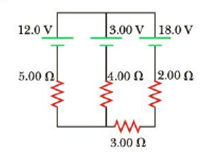Chapter 18, Problem 31P

Chapter
Section
Textbook Problem

Find the potential difference across each resistor in Figure P18.31.Figure P18.31

To determine
The potential difference across in each resistor.

Explanation

Given Info:

Resistance R1 is 5.00Ω , Resistance R2 is 4.00Ω , Resistance R3 is 3.00Ω , Resistance R4 is 2.00Ω , The expression for I2 is 3.00V0.800ΩI3 , the expression for I1 is 1.80V0.800ΩI3 , the current in the circuits I1 is 0.324A , I3 is 1.846A , I2 is 1.524A , Resistance R1 is 5.00Ω , Resistance R2 is 4.00Ω , Resistance R3 is 3.00Ω , Resistance R4 is 2.00Ω .

Explanation:

Voltage ε1 is 12.0V , Voltage ε2 is 3.00V , Voltage ε3 is 18.0V

The below figure shows the direction of current flow in the circuit.

• ε1,ε2,ε3 is voltage in the circuits
• R1,R2,R3,R4 is corresponding resistance in the circuits
• I1,I2,I3 is corresponding current in the circuits

Formula to calculate the expression for current by Kirchhoff’s voltage law

From the Left loop

ε1ε2R1I1R2I3=0

Substitute 5.00Ω for R1 , 4.00Ω for R2 , 3.00V for ε2 12.0V for ε1 in the above equation to find expression for I1

12.0V3.00V5.00ΩI14.00ΩI3=09.00V5.00ΩI14.00ΩI3=05.00ΩI1=9.00V4.00ΩI3I1=1.80V0.800ΩI3 (I)

From the right loop

ε3ε2R2I3(R3+R4)I2=0

Substitute 4.00Ω for R2 , 3.00Ω for R3 , 2.00Ω for R4 , 12.0V for ε2 , 18.0V for ε3 in the above equation to find expression for I1

18.0V3.00V4.00ΩI3(3.00Ω+2.00Ω)I2=015.0V4.00ΩI25.00ΩI2=05.00ΩI2=15.0V4.00ΩI3I2=3.00V0.800ΩI3 (II)

Conclusion:

Thus, from the Left loop the expression I1 is 1.80V0.800ΩI3 and from the right loop the expression I2 is 3

Still sussing out bartleby?

Check out a sample textbook solution.

See a sample solution

The Solution to Your Study Problems

Bartleby provides explanations to thousands of textbook problems written by our experts, many with advanced degrees!

Get Started